# JAC Class 9 Maths Solutions Chapter 14 Statistics Ex 14.2

Jharkhand Board JAC Class 9 Maths Solutions Chapter 14 Statistics Ex 14.2 Textbook Exercise Questions and Answers.

## JAC Board Class 9th Maths Solutions Chapter 14 Statistics Ex 14.2

Page-245

Question 1.
The blood groups of 30 students of Class VIII are recorded as follows:
A, B, O, O, AB, O, A, O, B, A, O, B, A, O, O
A, AB, O, A, A, O, O, AB, B, A, O, B, A, B, O.
Represent this data in the form of a frequency distribution table. Which is the most common, and which is the rarest, blood group among these students?
The frequency means the number of students having same blood group. We will represent the data in table:

 Blood Group Number of Students (Frequency) A 9 B 6 O 12 AB 3 Total 30

Most common Blood Group (Highest frequency): O
Rarest Blood Group (Lowest frequency): AB

Question 2.
The distance (in km) of 40 engineers from their residence to their place of work w ere found as follows:

 5 3 10 20 25 11 13 7 12 31 19 10 12 17 18 11 32 17 16 2 7 9 7 8 3 5 12 15 18 3 12 14 2 9 6 15 15 7 6 12

Construct a grouped frequency distribution table with class size 5 for the data given above taking the first interval as 0-5 (5 not included). What main features do you observe from this tabular representation?
The given data is very large. So, we con-struct a group frequency of class size 5. Therefore, class interval will be 0-5, 5-10, 10-15, 15-20 and so on. The data is represented in the table as: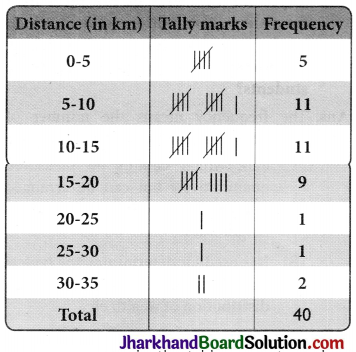The classes in the table are not overlap¬ping. Also, 36 out of 40 engineers have their house below 7. 20 km of distance.

Question 3.
The relative humidity (in %) of a certain city for follows: a month of 30 days was as

 98.1 98.6 99.2 90.3 86.5 95.3 92.9 96.3 94.2 95.1 89.2 92.3 97.1 93.5 92.7 95.1 97.2 93.3 95.2 97.3 96.2 92.1 84.9 90.2 95.7 98.3 97.3 96.1 92.1 89

(i) Construct a grouped frequency distribution table with classes 84 – 86, 86 – 88, etc.
(ii) Which month or season do you think this data is about?
(iii) What is the range of this data?
(i) The given data is very large. So, we construct a group frequency of class size 2. Therefore, class interval will be 84-86, 86-88, 88-90, 90-92 and so on. The data is represented in the table as below:

 Relative humidity (in %) Frequency 84-86 1 86-88 1 88-90 2 90-92 2 84-86 1 86-88 1 88-90 2 90-92 2 92-94 7 94-96 6 96-98 7 98-100 4 Total 30

(ii) The humidity is very high in the data which is observed during rainy season. So, it must be rainy season.
(iii) Range of data = Maximum value of data – Minimum value of data = 99.2 – 84.9 = 14.3%Question 4.
The heights of 50 students, measured to the nearest centimetres, have been found to be as follows:

 161 150 154 165 168 161 154 162 150 151 162 164 171 165 158 154 156 172 160 170 153 159 161 170 162 165 166 168 165 164 154 152 153 156 158 162 160 161 173 166 161 159 162 167 168 159 158 153 154 159

(i) Represent the data given above by a grouped frequency distribution table, taking the class intervals as 150-155,155-160, etc. soon.
(ii) What can you conclude about their heights from the table?
(i) The data with class interval 150-155, 155-160 and so on is represented in the table as:

 Height (in cm) No. of Students (Frequency) 150-155 12 155-160 9 160-165 14 165-170 10 170-175 5 Total 50

(ii) From the given data, it can be concluded that 35 students i.e. more than 50% are shorter than 165 cm.

Question 5.
A study was conducted to find out the concentration of sulphur dioxide in the air in parts per million (ppm) of a certain city. The data obtained for 30 days is as follows: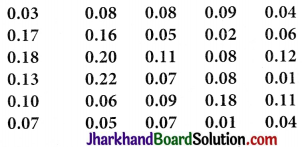(i) Make a grouped frequency distribution table for this data with class intervals as 0.00-0.04, 0.04-0.08, and so on.
(ii) For how many days, was the concentration of sulphur dioxide more than 0.11 parts per million?
(i) The data with class interval 0.00 – 0.04, 0.04 – 0.08 and so on is represented in the table as:

 Concentration of sulphur dioxide in air (in ppm) Frequency 0.00 – 0.04 4 0.04 – 0.08 9 0.08 – 0.12 9 0.12-0.16 2 0.16-0.20 4 0.20 – 0.24 2 Total 30

(ii) 2 + 4 + 2 = 8 days have the concentration of sulphur dioxide more than 0.11 parts per million.

Page-246

Question 6.
Three coins were tossed 30 times simultaneously. Each time the number of heads occurring was noted down as follows: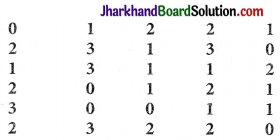Prepare a frequency distribution table for the data given above.
The frequency distribution table for the data given above can be prepared as follows:

 Number of Heads Frequency 0 6 1 10 2 9 3 5 Total 30Question 7.
The value of π upto 50 decimal places is given below:
3.14159265358979323846264338327950288419716939937510
(i) Make a frequency distribution of the digits from 0 to 9 after the decimal point.
(ii) What are the most and the least frequently occurring digits?
(i) The frequency is given as follows:

 Digits Frequency 0 2 1 5 2 5 3 8 4 4 5 5 6 4 7 4 8 5 9 8 Total 50

(ii) The digit having the least frequency occurs the least and the digit with highest frequency occurs the most. 0 has frequency 2 and thus occurs least frequently while 3 and 9 have frequency 8 and thus occur most frequently.

Question 8.
Thirty children were asked about the number of hours they watched TV programmes in the previous week. The results were found as follows: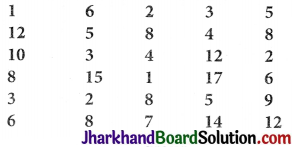(i) Make a grouped frequency distribution table for this data, taking class width 5 and one of the class intervals as 5-10.
(ii) How many children watched television for 15 or more hours a week?
(i) The distribution table for the given data, taking class width 5 and one of the class intervals as 5-10 is as follows:

 Number of Hours Frequency 0 – 5 10 5 – 10 13 10 – 15 5 15 – 20 2 Total 30

(ii) We observed from the given table that 2 children watched television for 15 or more hours a week.

Question 9.
A company manufactures car batteries of a particular type. The lives (in years) of 40 such batteries were recorded as follows: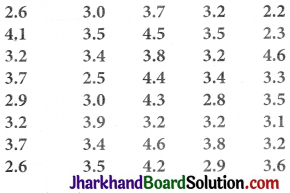Construct a grouped frequency distribution table for this data, using class intervals of size 0.5 starting from the interval 2-2.5.